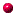Chapter 3

Constitutive ModelsRelations between Stress and Strain

3.4 Generalized Hooke’s lawelastic materials subjected to small stretches but large rotations

Recall that the stress-strain law for an anisotropic, linear elastic material (Sect 3.1) has the formwhereis stress (any stress measure you like),is the infinitesimal strain, andis the tensor of elastic moduli.This stress-strain relation can only be used if the material is subjected to small deformations, and small rotations.  This is partly because the infinitesimal strainfor a finite rotationso the law predicts that a nonzero stress is required to rotate a solid.

There are some situations where a solid is subjected to small shape changes, but large rotations.  For example, the picture shows a long slender beam bent into a circle by moments applied to its ends.  The strains in the beam are of order, where h is the thickness of the beam and R is its curvature.   The ends of the beam have rotated through a full 90 degrees, however.   The linear elastic constitutive equations would not predict the correct stress in the beam.It is easy to fix this problem: provided we choose a sensible (nonlinear) strain measure, together with the appropriate work-conjugate stress measure, we can still use a linear stress-strain relation.  To make this precise, suppose that a solid is subjected to a displacement field.  DefineThe deformation gradient and its jacobianThe Lagrange strainThe Eulerian strainThe rotation tensor (see 2.1.13 for the best way to compute R in practice)The Cauchy (“true”) stress, defined so thatThe Material stress (work conjugate to Lagrange strain)The Material stress-Lagrange strain relation can be expressed aswhereis the tensor of elastic moduli for the material with orientation in the undeformed configuration.  This is identical to the stress-strain relation for a linear elastic solid, except that the stress measure has been replaced by Material stress, and the strain measure has been replaced by Lagrange strain. You can therefore use all the matrix representations and tables of data given in Section 3.1 to apply the constitutive equation. The Cauchy (“true”) stress can be computed from the material stress asThe Cauchy stress-Eulerian strain relation: Alternatively, the stress-strain relation can be expressed in terms of stress and deformation measures that characterize the deformed solid, aswhereis the tensor of elastic moduli for the material with orientation of the deformed configuration.  This tensor is related tobyFor the special case of an isotropic material with Young’s modulus E and Poisson’s ratio(c) A.F. Bower, 2008
This site is made freely available for educational purposes.
You may extract parts of the text
for non-commercial purposes provided that the source is cited.
Please respect the authors copyright.